# SAT II Math II : Solving Linear Functions

## Example Questions

### Example Question #1 : Solving Functions

If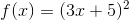, what must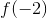be?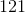Explanation:

Replace the value of negative two with the x-variable.

There is no need to use the FOIL method to expand the binomial.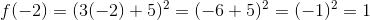The answer is:### Example Question #2 : Solving Functions

Let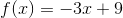.  What is the value of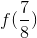?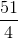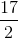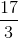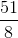Explanation:

Substitute the fraction as.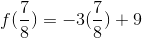Multiply the whole number with the numerator.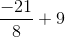Convert the expression so that both terms have similar denominators.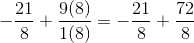The answer is:### Example Question #3 : Solving Functions

If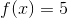, what must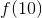be?Explanation:

A function of x equals five.  This can be translated to: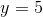This means that every point on the x-axis has a y value of five.

Therefore,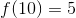.

The answer is: